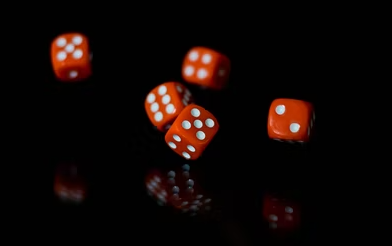## Rolling Dice

During a board game night, your team rolls 5 dice, one die at a time. What is the probability that the opposing team rolls the same numbers in the exact same order?Hint
Each of the 5 dice rolls could produce 6 different numbers, so there are $$6^{5}$$ different arrangements.
Hint 2
For the first dice, there is a 1/6 chance of matching.
Each of the 5 dice rolls could produce 6 different numbers, so there are $$6^{5}$$ different arrangements. For the first dice, there is a 1/6 chance of matching. The same is true for all subsequent dice rolls.
$$P=\frac{1}{6}\times \frac{1}{6}\times \frac{1}{6}\times \frac{1}{6}\times \frac{1}{6}=1/6^5$$$$$1/6^5$$$